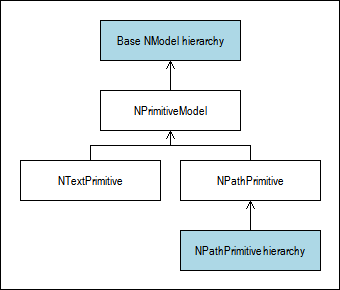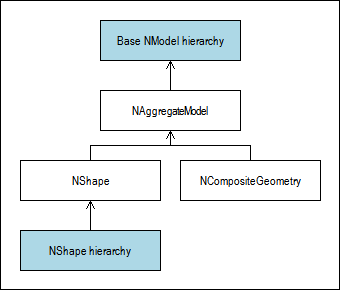﻿ Models
Nevron .NET Vision Documentation
Models

Models are transformable elements (derived from the NTransformableElement class) and as such host some transformable content, whose definition is not affected by the transformations applied to the element. Models are used for the hierarchical modeling of complex real world abstractions (see Coordinate Systems for more information).

In the DOM, models are represented by an instance of the NModel abstract class. In general there are only two types of models - primitive models and aggregate models. Aggregate models are assembled from other primitive and/or aggregate models. They use the following class hierarchy:Common model features

The NModel class adds support for the following common features shared by all models:

• Model Bounds - the model bounds define a rectangle, which bounds the model content. By design you can only get the model bounds via the ModelBounds property. The model bounds are always specified in the model (local) coordinate system. The model bounds projection in the scene coordinate system is in this documentation often referred as rotated bounds. The model bounds help you treat each model as a box.
• Scene Bounds - models implement the INBoundable interface to indicate that they have controllable bounds in scene coordinates. The bounds of a model are computed by calculating the bounds of the model bounds corners projection in scene coordinates. Changing the model scene bounds actually changes the model local transformation (not the model definition).
• Pin Point - the pin point is a point, whose position is aligned to the model bounds. The alignment of the pin point is controlled by the PinAlignment property. You can get and set the pin point in model and scene coordinates via the ModelPinPoint and PinPoint properties respectively. The set implementation of both properties will alter the pin alignment.

The pin point is used as default anchor (pivot) point in many transform operations. It is also used to define the relative location of a model in respect to its aggregate model.
• Start and End Points - each model has start and end points, which gives the model a certain direction. The start and end points of a model are exposed in model and scene coordinates through the following properties of each model: StartPoint, EndPoint, ModelStartPoint and ModelEndPoint.

By default start and end points of a model are aligned to the left-middle and right-middle sides of the model bounds. Also by default setting the start or end points will scale the model in the direction of the start-end point vector and rotate the model around the opposite point. Certain models can provide different implementation for their start and end points - for example the start and end points of a bezier curve primitive modify the bezier curve model definition.
• Logical Line - since each model has start and end points, you can logically treat it as a line (or curve) connecting these points. A logical line always starts from the start point and ends at the end point. It's length can be obtained from the Length property.

You can obtain a point along the line length, which corresponds to a percent value with the help of the GetLengthLocation method. You can also obtain the tangent angle (the slope of the line) along the length, which corresponds to a percent value with the help of the GetLengthOrientation method.

The logical line of a model can be reversed with the help of the Reverse method. Reversing the logical line of a model always causes the model to swap its' start and end points. In the case of a 1D shape this operation also forces the shape to swap the ports to which the start and end plugs are currently connected.

By default the logical line of a model is a straight line connecting the start and end points. The logical line of certain models however is a curve - for example bezier curve primitives use a logical line, which follows the bezier curve geometry.
• Contour  - each model can be treated as a contour. You can intersect the contour with a vector and get the nearest intersection point (if any). This is done with the help of the GetNearestContourLocation method.

By default the contour of a model is a polygon connecting the model bounds corners. The contour of certain models however is bound to the geometry they represent - for example the contour of an ellipse primitive is the ellipse rim.
• Mouse Event Handling - models implement the INMouseEventHandler interface, which is used by views to delegate mouse events to the model. The default implementation fires the respective mouse event for the model. If the event was not handled it bubbles the event to the previous INMouseEventHandler implementor in the scene hierarchy.
• Keyboard Event Handling - models implement the INKeyboardEventHandler interface, which is used by views to delegate keyboard events to the model if it is the currently focused element (controlled by the FocusedElementUniqueId property of the NDrawingDocument class). The default implementation fires the respective keyboard event for the model. If the event was not handled it bubbles the event to the previous INKeyboardEventHandler implementor in the scene hierarchy.
• Drag Drop Event Handling - models implement the INDragDropEventHandler interface, which is used by views to delegate drag-drop events to the model. The default implementation fires the respective drag - drop event for the model. If the event was not handled it bubbles the event to the previous INDragDropEventHandler implementor in the scene hierarchy.
Primitive models

Primitive models are leaf elements derived from NModel, which host the content of a single model. All primitive models derive from the base NPrimitiveModel abstract class. Currently there are only two types of primitive models - paths and texts. They use the following class hierarchy:Common for all primitive models is that they all contain a set of properties beginning with the "Model" prefix, which allow you to directly modify certain aspects of their model definition. For example: the ModelFirstControlPoint property of the NBezierCurvePath primitives controls the position of the bezier curve first control point in model coordinates.

Aggregate models

Aggregate models are branch elements derived from NModel, which aggregate the content of other primitive and/or aggregate models. All aggregate models derive from the base NAggregateModel abstract class. Aggregate models use the following class hierarchy:Aggregate models share the following set of common features:

• Element Container - aggregate models implement the INDiagramElementContainer interface, which consistently exposes access to it's child and descendant elements. Note that aggregate models are not composites - it is up to derived classes to provide the hierarchical structure of an aggregate model.
• Aggregated Models - the models aggregated by an aggregate model may not be it's direct children. That is why derived classes must implement the GetAggregatedModels abstract protected method in order to specify the models, which should be aggregated by the aggregate. For example: for a NCompositeShape this method will return a list of all shape primitives, for a NGroup this method will return a list of the shapes, which are contained in the group.
• Aggregated Model Bounds - since aggregate models do not have their own model definition, their model bounds are computed by calculating the union of the model bounds of the aggregated models, projected in the local coordinate system of the aggregate. Because of that the model bounds of an aggregate model may not always be up to date. This is sometimes a desired effect, but there are also cases in which it is not.

You can force an update of the model bounds with either custom or exact bounds. This is done with the help of the two overrides of the UpdateModelBounds method. For example:
C#
Copy Code
```// force the model bounds to be the exact bounding of the aggregated models
aggregateModel.UpdateModelBounds();
// force the model bounds to be some custom rectangle
aggregateModel.UpdateModelBounds(new NRectangleF(10, 10, 20, 20));
```
Visual Basic
Copy Code
```' force the model bounds to be the exact bounding of the aggregated models
aggregateModel.UpdateModelBounds()
' force the model bounds to be some custom rectangle
aggregateModel.UpdateModelBounds(New NRectangleF(10, 10, 20, 20))
```
You can also instruct an aggregate model to automatically update its model bounds, whenever the aggregated models have changed. This is done with the help of the AutoUpdateModelBounds property.
• Painting - aggregate models implement the INPaintable interface. The implementation delegates the painting to the aggregate children.
• Hit Tests - an aggregate model is considered hit, if one of it's aggregated models is hit.
Model Scaling

The only meaningful transform operation, which can be applied to the content of a model is scaling. Model scaling is different from transformation based scaling, since it directly scales the model definition (e.g. alters the models definition, not the transformation of the model). You can perform model scaling with the help of the ScaleModel method of the NModel class.

For a primitive model this will always scale the model definition of this model. For example: calling the ScaleModel method of a NPolygonPath primitive will scale the model points of the polygon.

Since an aggregate model does not have a model definition of it's own, this operation must somehow resize the aggregated models. The actual way in which an aggregated model is resized depends on the value of it's ResizeInAggregate property (accepts values from the ResizeInAggregate enumeration). If this value is set to UseAggregateSettings the model will be resized in accordance with the aggregate model ResizeAggregatedModels property (accepts values from the ResizeAggregatedModels enumeration).

Although there are a lot of resize modes, they actually specify two aspects of the resize, which must be performed - the type of scaling and whether the model should be repositioned after scaling.

In general there are four type of scaling:

• Affine Scale - in affine scaling the aggregate will alter the model local transformation (i.e. the model will be transformed). Affine scaling can be applied in both the X and Y aggregate resize directions. If the model is rotated inside its aggregate this can skew the model in the scale direction.
• Cartesian Scale - in Cartesian scaling the aggregate will proportionally scale the model in its local X and Y resize direction - i.e. it will call its ScaleModel method. The effect of Cartesian scaling is that if the model is rotated inside its aggregate this operation will not skew it, or in other words the model will preserve its orthogonality).
• Scale 1D - in 1D scaling the aggregate will scale only the start and end points of the model.
• No Scale - the aggregate will not scale the model.

Reposition refers to the ability to specify whether the model pin point must preserve its location relative to the resized aggregate model bounds (i.e. whether the model must be "pinned" to its relative location in the aggregate).

Examples:

• ResizeInAggregate.CartesianScaleAndReposition - means apply Cartesian scale to the model and preserve its pin point relative to the aggregate model bounds.
• ResizeInAggregate.AffineScaleXRepositionY - means apply affine scale to the model in the X direction and preserve its pin point Y position relative to the aggregate model bounds.
• ResizeInAggregate.RepositionOnly - means do not scale the model, but preserve it's pin point relative to the aggregate model bounds.
Related Examples
Windows Forms: Document Object Model - Groups - Group Resize